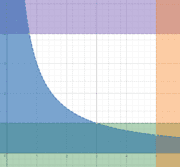# Volume Integration Around Non-Coordinate Axis

## Homework Statement

Find the volume of the solid generated by revolving the region bounded by the graphs of the equations about the line x = 5. (Use disk method)

$$xy = 3, y = 1, y = 4, x = 5$$

## Homework Equations

[/B]
The formula using for disk method is of the form:

$$\pi \int (r(x/y))^2*(dx/y)$$

## The Attempt at a Solution

[/B]
So first I graphed this out, and came up with a region, like so (represented by the white region):In disk method, when rotating around a vertical axis, the differential of dy is used.

Setting this integral up, our limits are y = 1, to y = 4, as given in the problem statement.

My main question is how do we represent the r in the disk method equation, when the axis is meant to be around x = 5, and not the y-axis.

The best I could think of would be:

$$\pi \int_{1}^{4} (\frac {y} {3} + 5)^{2} dy$$

However, something tells me this isn't the right approach, because I'm not seeing the logic behind whether or not I should add, subtract, or subtract from the x = 5.

Thank you.

SteamKing
Staff Emeritus
Homework Helper

## Homework Statement

Find the volume of the solid generated by revolving the region bounded by the graphs of the equations about the line x = 5. (Use disk method)

$$xy = 3, y = 1, y = 4, x = 5$$

## Homework Equations

[/B]
The formula using for disk method is of the form:

$$\pi \int (r(x/y))^2*(dx/y)$$

## The Attempt at a Solution

[/B]
So first I graphed this out, and came up with a region, like so (represented by the white region):In disk method, when rotating around a vertical axis, the differential of dy is used.

Setting this integral up, our limits are y = 1, to y = 4, as given in the problem statement.

My main question is how do we represent the r in the disk method equation, when the axis is meant to be around x = 5, and not the y-axis.

The best I could think of would be:

$$\pi \int_{1}^{4} (\frac {y} {3} + 5)^{2} dy$$

However, something tells me this isn't the right approach, because I'm not seeing the logic behind whether or not I should add, subtract, or subtract from the x = 5.

Thank you.
Remember, r(y) is the distance measured from the curve xy = 3 to the line x = 5. Examining your graph should tell you what r(1) must be. I don't think that's what the integrand in your integral indicates.

SammyS
Staff Emeritus
Homework Helper
Gold Member

## Homework Equations

[/B]
The formula using for disk method is of the form:$$\pi \int (r(x/y))^2*(dx/y)$$
I suspect that the formula you gave for the disk method is to be interpreted as a general formula in which you will use only x or only y , depending upon whether the axis is horizontal or is vertical .

Use ##\displaystyle \ \ \pi \int (r(x))^2\ dx\ \ ## or ##\displaystyle \ \ \pi \int (r(y))^2\ dy\ \ ## as appropriate.

So, you could interpret the distance as ## 5 - x ##? and thus make the ## r = 5 - \frac 3 y ## ?

The integral would then become ## \pi \int_{1}^{4} (5 - \frac {3} {y} )^{2} dy ## ?

SammyS
Staff Emeritus